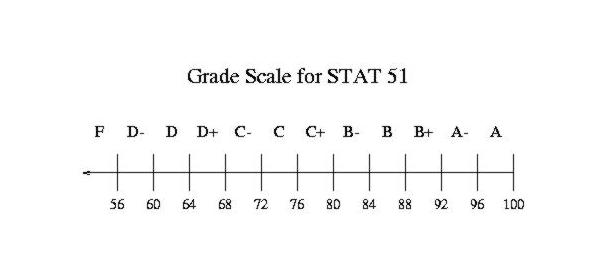# Final Exam Scores and Semester Grades

```
Important inferences about the class of STAT1051 can be made by inspecting
the following histograms. Note the symmetry of the distribution of the
Final Exam Scores. The distribution is centered on the class median.Full Screen of Histogram of Final Exam Scores

The 95% CI about the mean for the Spring 2019 Final Exam is: (78, 84).
The mean and median for the Final Exam are 81 and 84.
The 95% CI about the mean for the semester grades is: (85, 90).
The mean and median for the semester grades are 88 and 89, respectively.
The top six scores on the Final Examination are: 100 99 98 97 97 96
The top six semester scores are: 104 103 103 102 101 99

Because the quiz average, Mid-term Exam, and Final Exam carry different
applied to them. But suppose the Final Exam were to be graded according tothen the grades in the histogram of the Final Exam Scores can serve as a
simple though biased indication of how well one did on the Final Exam in termsFull Screen of the Histogram of Semester Scores

A noteworthy relationship can be observed in the plot of the Semester
Scores and the average Quiz score. The plot shows that a linear relationship
exits between Semester Scores and the average Quiz score. We learned
in STAT1051 that by means of the method of least squares, it is possible
to test the hypothesis that the slope equals zero versus the alternative
that it does not equal zero. The F statistic, as you might imagine, is
very large. In other words, there is evidently an inherent relation
between doing the quizzes well and getting a good semester grade.Full Screen of the Relation between Semester Scores and Quiz Average

In fact, the relation is so strong that it can be exhibited on the first day
of class to convince everyone in STAT1051 of the importance of doing the
homework from which the quizzes are derived. Notice that, from the equation
of the fitted least squares line, one can reckon a semester grade given
the current average quiz score.